# High Side Driver Design Using PMOS with Resistive Load

High side driver design using PMOS is an easy approach on driving a high side load. High side driver is the term used because once the switch turns on, it will connect the load to the circuit supply to complete the current path. A load is not limited to resistors, it can also an inductive such as relay and lamp. In this article we will concentrate with the resistive load.

### Circuit Schematic for High Side Driver Design Using PMOS

Figure 1 below is the circuit schematic of the high side driver we want to design. Rlimit is not necessary all the time. It is a must when using a power MOSFET in switching converter. Power MOSFET has high input capacitance. During startup this capacitance act as a short circuit so the initial peak current  is huge and may damage the device that drive the gate of the MOSFET. Another reason of adding this Rlimit to power MOSFET in switching converter is to minimize the dv/dt. Too high dv/dt will damage the MOSFET especially at higher temperature operation. In small signal MOSFET, this is not needed.

In using power MOSFET in switching converters, the value of Rlimit is can be set according to the recommendation of the MOSFET vendor. This is usually less than 100Ω.

R1 is a must to include. This will ensure that MOSFET will stay off when the input voltage is removed or left float. R1 is can be set to 10kΩ or higher.

For PMOS, the gate voltage must be less than the source voltage. The difference must be higher than the gate threshold voltage of the MOSFET.

In above circuit, Vin is can be coming from a microcontroller or digital signal controllers/processors if:

`a.) MOSFET is small signal type`

If the MOSFET is only a small signal, the input capacitance is not a problem and the danger of damaging the microcontroller or digital ICs due to large inrush current is not possible.

`b.) The circuit Vdd is only within the Vcc level of the microcontroller or digital ICs`

When the level of Vdd is above the Vcc level of the microcontroller or digital ICs which are commonly 5V, the MOSFET cannot be driven by microcontroller or digital ICs. The very reason is that you cannot turn off the MOSFET. In order to turn off a PMOS, the gate must be equal or higher than the source. Supposing the level of Vdd in the above circuit is 12V and Vin is derive from a microcontroller with a pin voltage of 5V, there’s no way to turn off the MOSFET. The gate is always lower than the source (12V-5V).

Below are the steps to follow for high side driver design using PMOS.

### Step 1: Select a MOSFET

In selecting a MOSFET you need to know or have estimate on the maximum load current that will flow to the MOSFET. Compare this load current to the drain current rating of the MOSFET. If the estimated load current is 500mA, better use a MOSFET with a 1A capability to provide margin. A 1 ampere rated MOSFET is not anymore 1A when the ambient temperature is higher than 25’C.

Select a MOSFET that has a drain voltage capability higher than the maximum level of Vdd in the circuit. As a rule of thumb, utilize only up to 70%. Say the maximum voltage the device can handle is 30V, the maximum level of Vdd must only be 21V.

Also consider the gate threshold voltage in the selection of the device. If you are going to drive the MOSFET gate with a microcontroller or digital ICs, the maximum gate threshold voltage of the MOSFET must be less than 5V (Vcc level of the microcontroller) with a margin.

Another thing to consider is the gate to source voltage maximum rating. PMOS usually has lower gate to source voltage rating than NMOS, so make sure that the maximum gate voltage is not exceeding this level. So if you want to drive the gate with a microcontroller output, the PMOS +/-VGS must be higher than the microcontroller pin output.

### Step 2: Satisfy the VGS Threshold Requirement

In order to turn on a MOSFET, its gate threshold voltage must be satisfied. In order to fully saturate a MOSFET, its applied gate to source voltage must be significantly higher than the threshold level. In NMOS, the applied gate to source voltage is positive which means that the gate is higher than the source. This is why NMOS is easier to use as a low side switch or driver. To know how to design a low side switch using NMOS, read THIS.

On the other hand, in PMOS the gate to source voltage must be negative which means that the source is more positive than the gate for the MOSFET to turn on.

Supposing we are using here Si1013 part, the maximum threshold voltage needed is not defined; only the minimum one which is -0.45V as below data. We will not use the minimum level as reference. In this case you can contact the vendor and ask for the maximum value or simply find another MOSFET.On the datasheet of Si1013 part, there is a graph between gate charge and gate to source voltage. We can see based from this graph that the maximum gate voltage needed to exit the miller charging state is around 1.3V (actually this is negative since PMOS)  . (The flatness of the curve is the time the miller capacitor is charging. Once the miller capacitor is full, the gate voltage will go to the set level.)So the minimum gate to source voltage of the circuit above must be more negative than -1.3V. Considering the level of Vdd is 5V and the minimum level of Vin is 0V (coming from a microcontroller unit), the applied gate to source voltage is then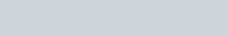The applied gate to source voltage is more negative than the maximum gate to source threshold voltage. The MOSFET can saturate.

To turn off the MOSFET, the level of Vin in above schematic must be equal or greater than the level of Vdd. If we are to assume here to use microcontroller as the gate driver, the high output of microcontroller is 5V and the level of Vdd is also 5V; so the MOSFET can turn off.

### Step 3: Check the Drain Current

With the selected MOSFET and the load, check the drain current using below equation.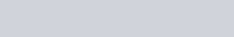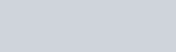RDSon is the on-state resistance of the MOSFET. Supposing we are using Si1013 part, the RDSon is 0.8Ω to 1.2Ω taken at 25’C at VGS of -4.5V. We are selecting the data at -4.5V because our applied VGS is -5V and this is the closest value.The above data are taken at 25’C ambient temperature only. If the circuit is exposed to ambient temperatures higher than 25’C, the RDSon may increase. In this case, we will get the relative value on the graph below.Supposing the maximum temperature the circuit is exposed to is 100’C, the resulting RDSon isIn checking the drain current our objective is to know the device stress. Thus, we can use the minimum value of the RDSon or make it zero to get the worst case. Considering the value of R1 is 1kΩ and the level of Vdd is 5V, the drain current is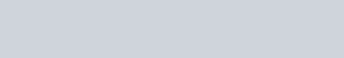The given drain current in the datasheet of Si1013 is 300mA maximum at 25’C.Above drain current rating is only a typical value. At 100’C operation, this drain current will de-rate. Some vendors include a graph of ambient temperature versus the drain current. So at 100’C ambient temperature, get the drain current and this is the capability of the device at this operating temperature. There is no such graph in the datasheet for this device (Si1013), so we can use the computation method.

For Small Signal MOSFETFor Power MOSFETWhere;

Tjmax is the maximum junction temperature of the MOSFET, Tamax is the maximum operating ambient temperature, Tcmax is the maximum case temperature of the MOSFET in actual use, Rthja is the thermal resistance from junction to ambient, Rthjc is the thermal resistance from junction to case and RDSon is the on state resistance.

We will apply above equation in Si1013 part. Si1013 is a small signal MOSFET so we are going to use the first equation. The Tjmax is 150’C, the Rthja is 833K/W for SC-75 package and our computed RDSon for 100’C operating ambient is 1.008 minimum.### Step 4: Check the Power Dissipation

Use below equation to check the power capability of the device and compare this to the computed power dissipation.For Si1013,The power dissipated  by the MOSFET is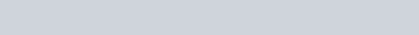The computed power dissipation is less than the capability of the device with a huge margin. Therefore there is no danger related to power stress.

This site uses Akismet to reduce spam. Learn how your comment data is processed.